## Section41.3Faraday's Flux Rule and Lenz's Law

Various experiments of Faraday show that an electricity is induced in a circuit when there is a changing magnetic field or if the loop is moving in a magnetic field. These effects are collectively called the electromagnetic induction.

Recall a simple circuit containing a voltage source as in Figure 41.3.1. The current in this circuit is driven by the electric field that gets set up in the wire by the voltage source. The work done by the voltage source in driving the current in the circuit per unit charge is called electromotive force (EMF), which is same as voltage there.

Faraday's experiments described in Section 41.1 show that a current can be induced in a circuit even when there is no voltage source. So, how that can be? Faraday reasoned that induced current must be due to an induced EMF in the circuit. Because, induced current was intimately connected to magnetic field, Faraday also reasoned that induced EMF must be somehow related to the magnetic flux.

Faraday explained all his experiments by a simple rule, often referred to as Faraday's flux rule. It states that induced EMF in a loop is equal to the rate at which magnetic flux through the loop changes. Sometimes this rule is also referred to as Faraday's law - we will reserve that phrase for a more general result to be described in Section 41.7.

Let us denote induced EMF by $\mathcal{E}_\text{ind}$ rather than symbol $V$ since we are dealing with dynamical quantity as opposed to static quantity of a voltage of a battery. According to Faraday's flux rule, average induced EMF during interval $t$ to $t+\Delta t$ is given by the rate of change of magnetic flux.

\begin{equation} \mathcal{E}_\text{ind,ave} = - \dfrac{\Delta \Phi_B}{\Delta t}.\label{eq-faraday-law-average-EMF}\tag{41.3.1} \end{equation}

You can see that the unit of $\Delta \Phi_B / \Delta t$ is actually volts, same as the unit of battery voltage. Thus, if at some instant, $\Delta \Phi_B / \Delta t$ was $12\text{ V}\text{,}$ then, the circuit will have current corresponding to a $12\text{ V}$ fictitious battery in the circuit. We will see examples of its applications below.

#### Subsubsection41.3.1.1Flux Rule for Uniform Magnetic Field

For uniform magnetic field and flat area we found above that $\Phi_{B} = B A \cos\,\theta\text{.}$ Therefore, a change in magnetic flux can occur in three ways:

1. $\Delta \Phi_B$ due to changing $B\text{,}$ i.e, changing strength of $\vec B\text{,}$

\begin{equation*} \Delta \Phi_{B,B} = (A \cos\,\theta )\Delta B, \end{equation*}
2. $\Delta \Phi_B$ due to changing $A\text{,}$ i.e, changing area in the loop through which field lines may go through,

\begin{equation*} \Delta \Phi_{B,A} = (B \cos\,\theta )\Delta A, \end{equation*}
3. $\Delta \Phi_B$ due to changing $\theta\text{,}$ i.e, relative orientation of $\vec B$ and area.

\begin{equation*} \Delta \Phi_{B,\theta} = -(B A \sin\,\theta )\Delta \theta, \end{equation*}

where you have $-\sin\,\theta$ since you need to take a derivative of cosine, when you are looking at changing orientation.

Pooling the three types of contributions you will get the net change in flux in time $\Delta t\text{.}$

\begin{equation} \Delta \Phi_B = \Delta \Phi_{B,B} + \Delta \Phi_{B,A} + \Delta \Phi_{B,\theta}.\tag{41.3.2} \end{equation}

Therefore, in the case of uniform magnetic field through a flat area, induced EMF will come from three sources.

\begin{equation} \mathcal{E}_\text{ind,ave} = - \dfrac{\Delta \Phi_{B,B}}{\Delta t} -\dfrac{\Delta \Phi_{B,A}}{\Delta t} - \dfrac{\Delta \Phi_{B,\theta}}{\Delta t}.\label{eq-faraday-law-average-EMF-three-sources}\tag{41.3.3} \end{equation}

Faraday's experiments described in Section 41.1 illustrate these mechanisms by which flux can change in a closed loop.

Direction of Induced Current. We will see below in Subsection 41.3.3 that Lenz's law is an easier way to glean the direction of induced current, but here we make the point that minus sign in Eq. (41.3.1) tells us the direction of the induced EMF, itself, which is the direction of the induced current.

Minus sign in Eq. (41.3.1) gives us the direction of induced current when we use right-hand rule to assign direction to the area of the loop as illustrated in Figure 41.3.2. If $\mathcal{E}_\text{ind,ave}$ is positive, then induced current will be in the direction of the area vector and if $\mathcal{E}_\text{ind,ave}$ is negative, then induced current is in the opposite direction.

Magnitude of Induced Current. Let $\mathcal{E}_\text{ind}$ be the induced EMF in a loop of wire that has resistance $R\text{,}$ then by Ohm's law we would get induced current $I_\text{ind}\text{.}$

\begin{equation} I_\text{ind} = \dfrac{1}{R}\,\mathcal{E}_\text{ind}.\tag{41.3.4} \end{equation}

So, how is $\mathcal{E}_\text{ind}$ related to moving conductor, moving magnet, or changing magnetic field? We will work formulas out in particular situations below and then give a complete answer in the end.

### Subsection41.3.2Flux Rule and Stacking Loops

Faraday used stacked coils to enhance the induced EMF in the loop. A long wire is twisted into coils and the ends of the wire are connected to complete the loop. We can now understand why coils enhance the electromagnetic effect.

Consider two circuits labelled $\mathcal{C}_1$ and $\mathcal{C}_2$ in Figure 41.3.3 which are made of identical material and have the same size loops. In the circuit $\mathcal{C}_1$ there is only one loop and in the circuit $\mathcal{C}_2$ there are four loops which are connected so that the induced current would flow parallel in all the loops. Note that the wire will have to be twisted to connect the ends so that current flows in the same direction in all the loops.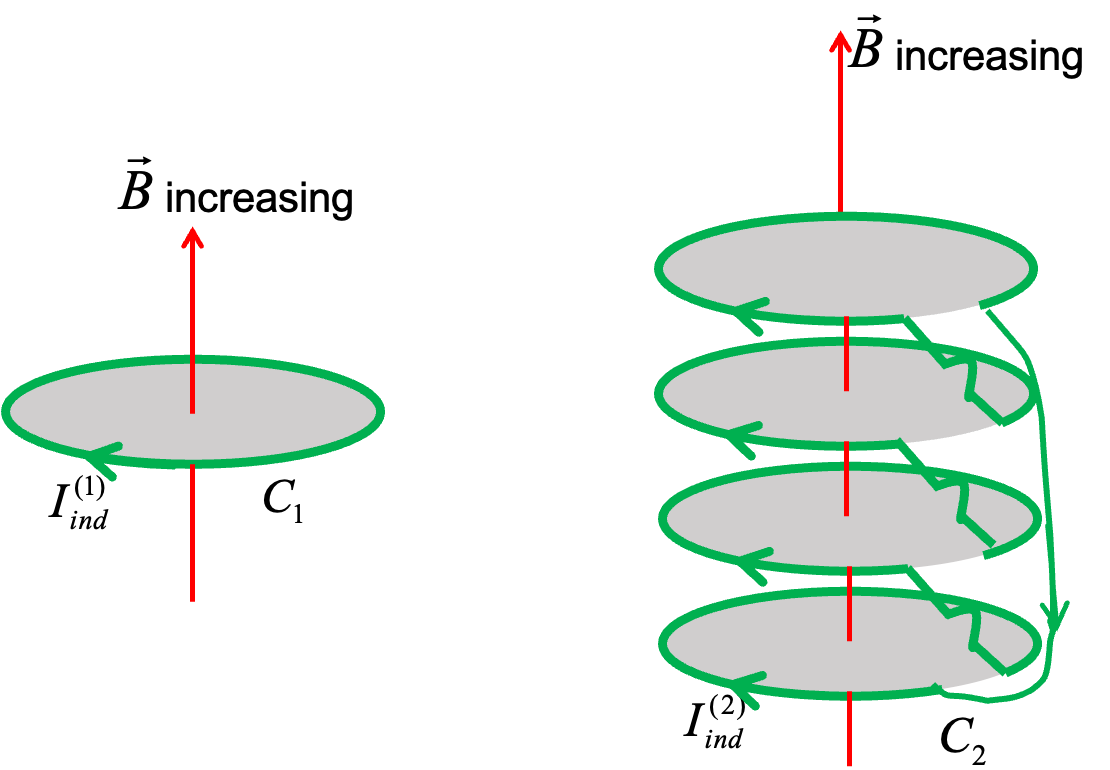Figure 41.3.3. Varying magnetic field induces four times as much EMF as in circuit $\mathcal{C}_2$ as in circuit $\mathcal{C}_1$ and hence four times as much current.

Suppose we place circuit $\mathcal{C}_2$ in an external magnetic field and then we vary the strength of the field.

Now, we ask: how do various quantities in the two circuits compare? In particular, we compare the rates of the change magnetic fluxes and the induced EMFs in the two circuits. Let us use superscripts $(1)$ and $(2)$ for the quantities in the two circuits.

We see that a simple surface can be attached to calculate the magnetic flux through the one-loop circuit $\mathcal{C}_1\text{,}$ but no simple surface can be attached to all the wires of circuit $\mathcal{C}_2$ due to the connecting wires on the side. In Figure 41.3.3, I have highlighted only the loop areas and omitted other areas around the loop that would be need for all areas enclosed by the entire circuit. These other areas are negligible in our case and it is okay to ignore them. With this assumption, we see that the magnetic flux through $\mathcal{C}_2$ is four times the magnetic flux through $\mathcal{C}_1\text{.}$

\begin{equation*} \left|\Phi_B^{(2)}\right| \approx 4\times \left|\Phi_B^{(1)}\right| \end{equation*}

We will now ignore the small error we make in neglecting the extra surface on the side of the loops and will write the relations as an equality below. Thus, the rate of change of the magnetic flux through $\mathcal{C}_2$ is four times that through $\mathcal{C}_1\text{.}$

\begin{equation*} \left|\dfrac{d\Phi_B^{(2)}}{dt} \right|= 4\times \left|\dfrac{d\Phi_B^{(1)}}{dt}\right|, \end{equation*}

which implies that the induced EMF in $\mathcal{C}_2$ would be four times that in $\mathcal{C}_1$

\begin{equation*} \mathcal{E}^{(2)} = 4\times \mathcal{E}^{(1)} \end{equation*}

### Subsection41.3.3Lenz's Law

When you examine the direction of the induced magnetic field by the induced current and compare it with the direction of the external magnetic field, you notice the following observation, known as Lens's law.

The flux of the magnetic field generated by the induced current always opposes the change in the magnetic flux through the loop. Lenz explained this by claiming that each closed circuit has an inertia of magnetic flux and develops induced current to oppose any change in its state.

It is easy to figure out the direction of induced current by applying Lenz's law. For instance, suppose external magnetic field was directed towards positive $z$ axis and it was increasing. In this case, the magnetic field of the induced current will be in the negative $z$ direction, as illustrated in Figure 41.3.4. On the other hand, if the magnetic field was decreasing, then, the magnetic field of the induced current will be in the positive $z$ direction.

From the required direction of the induced magnetic field, we can deduce the current that must have been induced by using Biot-avart's right-hand rule.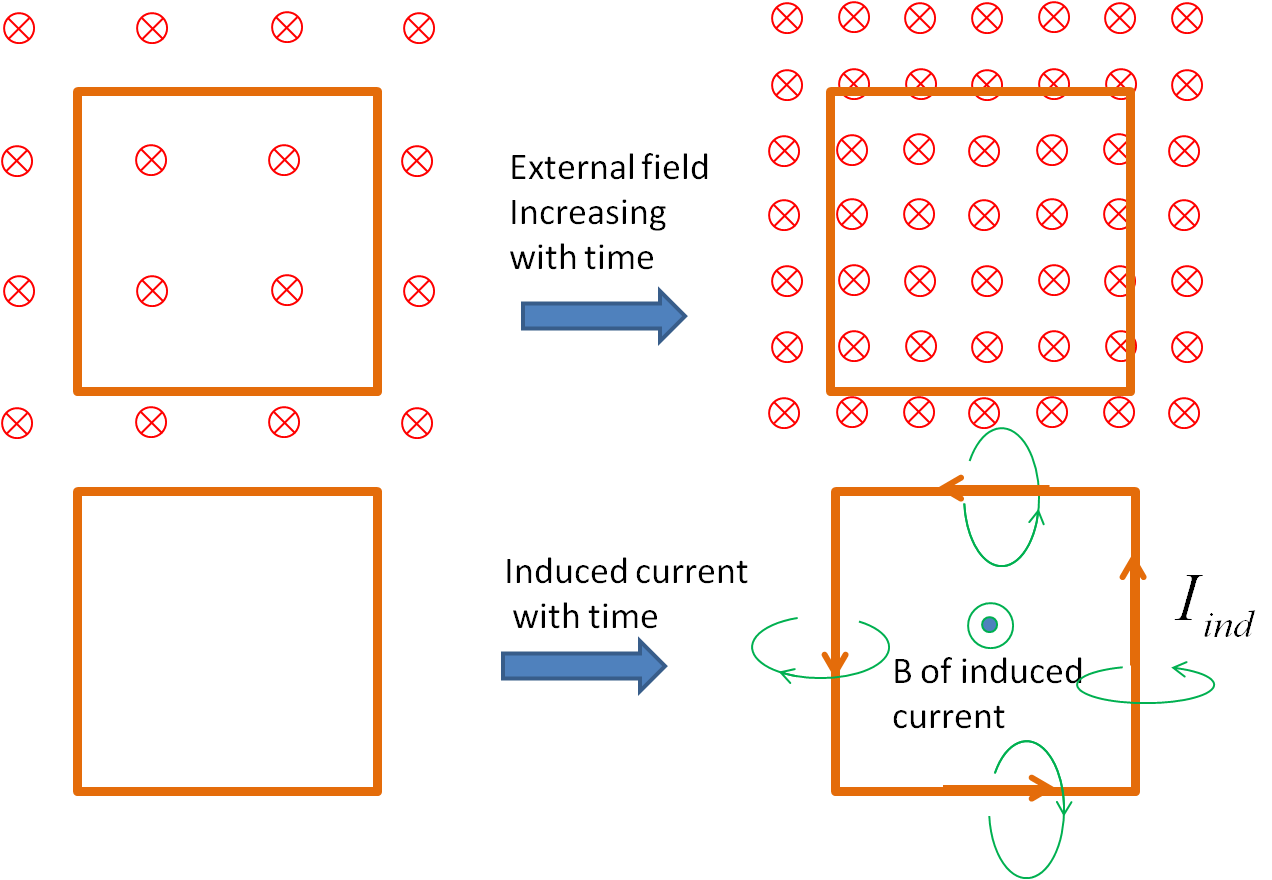Figure 41.3.4. As magnetic flux through the conducting loop in the direction into-the-page increases, the induced current in the loop develops, whose magnetic field must have the direction out-of-page, denoted by symbol $\bigotimes\text{,}$in the area of the loop to fight the increasing flux. To create magnetic field in $\bigotimes$ direction in the area of the loop, the induced current must be running counterclockwise by using Biot-Savart's right-hand rule.

Faraday's flux rule does not explain induced current in all stuations. For instance, Figure 41.3.5 shows an adaptation of Faraday's disc problem in a practical setting, where you need to go beyond flux rule and include Lorentz force on moving electrons to explain the development of induced current.

In Figure 41.3.5, a conducting disc is connected to a galvanometer with one contact at the shaft and the other at the edge of the disc so that the contacts rub the metal shaft and the metal edge when the disc rotates. A magnet is fixed to provide a magnetic field perpendicular to the surface of the disc.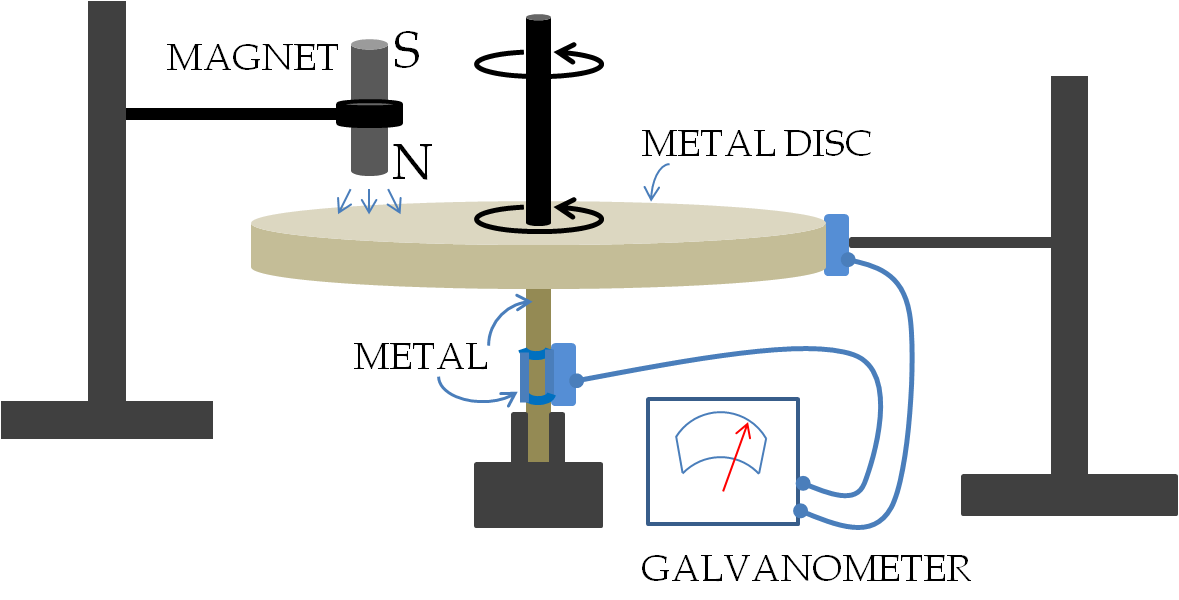Figure 41.3.5. A metal disc is rotated in the presence of a fixed magnet. When the disc rotates, there is no change in magnetic flux through any choice of line on the disc to complete the loop through the galvanometer. Yet there is an induced current through the galvanometer. The EMF for the current through the galvanometer can be understood in terms of radially pointed Lorentz force, $q \vec v\times \vec B\text{,}$ on the moving conduction electrons at the points on the disc with non-zero magnetic field. Adapted from Feynman's Lectures in Physics, Vol II.

First note that a unique circuit is not clear here since you can draw infinitely many lines on the disc between the contact at the edge and the contact at the shaft. The magnetic flux through any circuit you draw in space would not change with time. However, when the disc rotates a current is detected by the galvanometer.

A naive application of the flux rule gives us a contradiction here: the flux rule says that no flux change would mean no induced current. However, a closer look at the experimental setting shows that the magnetic force, $q\vec v\times\vec B\text{,}$ on the moving charges will cause a radial component of the velocity which will result in an overall drift of charges towards the shaft. The EMF in this setting develops neither from a changing magnetic field nor from a changing area of a loop or a changing orientation of a loop.

We can work out the induced EMF by Lorentz force as follows. Suppose electrons in the metal are moving with velocity $\vec v \text{,}$ then there would be magnetic force on conduction electrons along the wire, thus accelerating them perpendicular to their motion.

\begin{equation} \vec F_{m} = q \vec v \times \vec B.\tag{41.3.5} \end{equation}

This force on conduction electrons will cause drift motion in the conductor guided by the geometry of the conductor. Since accelerating electrons cannot leave the conductor, this results into an induced electric field in the conductor, which is equal to this force per unit charge.

\begin{equation} \vec E_\text{ind} = \dfrac{\vec F_{m} }{q} = \vec v_\perp \times \vec B.\tag{41.3.6} \end{equation}

Asssuming this field to have constant magnitude and flowing in the conducing loop of length $l\text{,}$ the EMF associated with this induced field will be

\begin{equation} \mathcal{E}_\text{ind} = - E l = - |\vec v_\perp \times \vec B| l .\label{eq-faraday-law-EMF-lorentz-force}\tag{41.3.7} \end{equation}

Use Lenz's law to determine the direction of induced current in each case. Here a circle with a dot, $\bigodot$ represents magnetic field pointed out-of-page and a circle with an x, $\bigotimes$ for a magnetic field pointed in-the-page, and lines are conductors.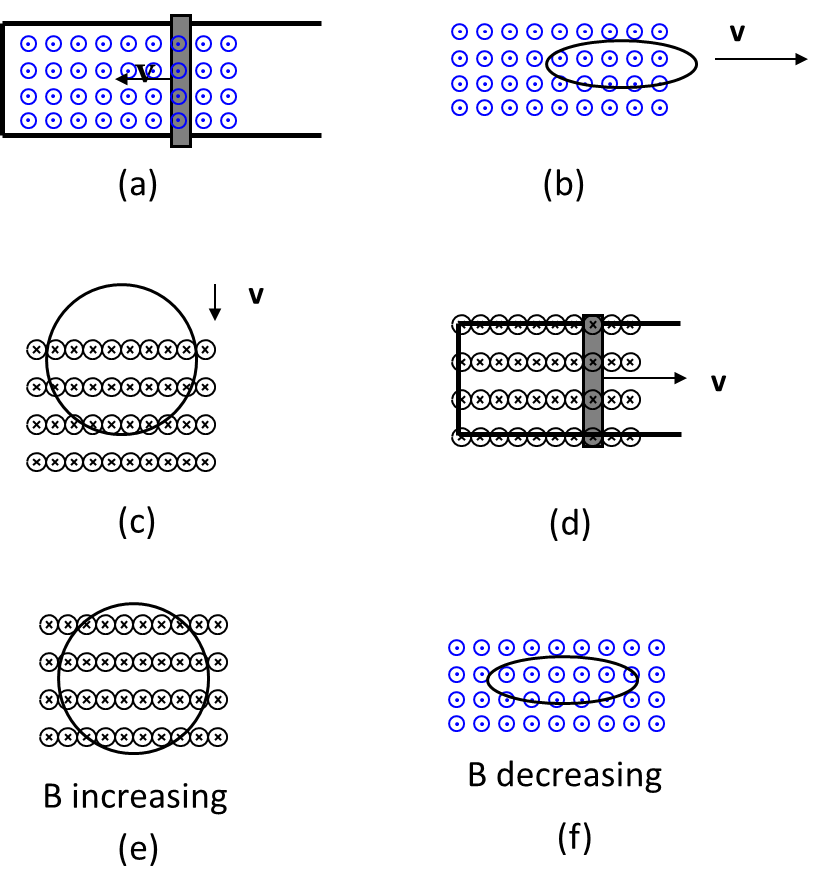Hint

Apply Lenz's law.

(a) up the rod, (b) counterclockwise, (c) clockwise, (d) counterclockwise, (e) counterclockwise, (f) counterclockwise.

Solution 1 (a)

(a) Magnetic flux from magnetic field in the direction out-of page decreases inside the loop. Hence the induced current must create more of magnetic field in the out-of-page direction. Therefore the induced current will flow up in the rod.

Solution 2 (b)

(b) Since the ring is leaving an area of magnetic field in the out-of page direction, the induced current in the ring must generate magnetic field in the out-of-page direction. Hence, induced current will be in the counterclockwise direction.

Solution 3 (c)

(c) The ring is moving so that magnetic flux from magnetic field in the in-the-page direction is increasing. Therefore the induce current will be creating magnetic field in the out-of-page direction so as to reduce the increase of in-the-page magnetic field. A current in the clockwise direction will be induced.

Solution 4 (d)

(d) As the bar moves, the loop encloses more of in-the-page magnetic field lines. Hence, the induced current will produce out-of-page magnetic field inside the space enclosed by the loop. Therefore, the induced current will run in a counterclockwise direction.

Solution 5 (e)

(e) When $B$ into the page is increasing, the magnetic flux through the ring for into-the-page will be increasing. The induced current will be in such a direction that the magnetic field of the induced current will be in the out-of page direction. Therefore, the direction of the induced current will be counterclockwise as shown.

Solution 6 (f)

(f) When $B$ out-of-page is decreasing, the magnetic flux through the ring for out-of-page will be decreasing. The induced current will be in such a direction that the magnetic field of the induced current will be in out-of page direction. Therefore, the direction of the induced current will be counterclockwise as shown.

A current is induced in a circular loop of radius 1.5 cm between two poles of a horseshoe electromagnet when the current in the electromagnet is varied. The magnetic field in the area of the loop is perpendicular to the area and has a uniform magnitude. If the rate of change of magnetic field is 10 T/s, find the magnitude and direction of the induced current if resistance of the loop is 25 $\Omega\text{.}$

Hint

Use flux rule in combination with Lenz's law.

$2.83\times 10^{-4} \ \textrm{A}\text{.}$

Solution

The magnitude of the induced current is found by dividing the induced EMF given by Faraday law.

\begin{equation*} I_{\textrm{ind}} = \dfrac{\left|d\Phi/dt \right|}{R_{\textrm{eq}}} = \dfrac{2\:\textrm{T/s}\times \pi (0.015\:\textrm{m})^2 }{5\:\Omega} = 2.83\times 10^{-4}\:\textrm{A}. \end{equation*}

The direction will be such that it will oppose the increase of magnetic flux as shown in Figure 41.3.14. The magnetic flux of the external field in the left to right direction is increasing. Therefore, induced magnetic field will be pointed to the left at points inside of the loop. The induced current will be in the direction shown.

Current in a long solenoid of radius 3 cm varies with time at a rate of 2 A/s. A circular loop of wire of radius 5 cm and resistance 2 $\Omega$ surrounds the solenoid. Find the electric current induced in the loop.

Hint

Use loop rule.

$7.1\ \mu \ \textrm{A}\text{.}$

Solution

Note that even though there is no magnetic field at the site of the outer wire, there is a no-zero magnetic flux through the area of the loop which is changing in time. Hence, a current will be induced in the outer wire given by Faraday law.

\begin{align*} I_{\textrm{ind}} \amp = \dfrac{\mathcal{E}}{R_{\textrm{outer loop}}} \\ \amp = \dfrac{\mu_0\:n\:A_{\textrm{sol}}}{R_{\textrm{outer loop}}}\:\dfrac{\Delta I_{\textrm{sol}}}{\Delta t}, \end{align*}

where $A_{\textrm{sol}}$ is the area of cross-section of the solenoid and $I_{\textrm{sol}}$ is the current in the solenoid at time $t\text{.}$ Putting in the numerical values we obtain

\begin{equation*} I_{\textrm{ind}} = 7.1\times 10^{-6}\:\textrm{A}. \end{equation*}

A long solenoid of radius a with n turns per unit length is carrying a time-dependent current $I(t) = I_0 \sin (\omega t)\text{,}$ where $I_0$ and $\omega$ are constants. The solenoid is surrounded by a wire of resistance $R$ that has two circular loops of radius $b$ with $b \gt a\text{.}$ Find the magnitude and direction of current induced in the outer loops at time $t=0\text{.}$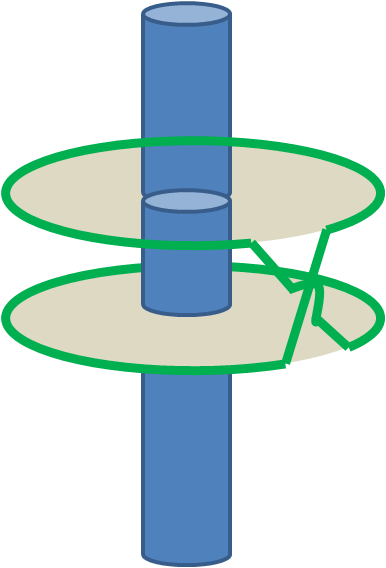Hint

Use flux rule to find the induced EMF first.

$2\mu_0 \pi a^2 I_0 n\omega/R\text{.}$

Solution

It is easier to calculate the magnetic flux of the magneitc field of the current in the solenoid through the outer loops since the magnetic field of the solenoid is constant.

\begin{equation*} \Phi_{\textrm{through outer loops}} = 2\times B_{\textrm{sol}}\times \pi a^2 = 2\times \mu_0\:n\:I_{\textrm{sol}}\times \pi a^2. \end{equation*}

Note the area over which the magnetic field of the solenoid is non-zero is only the inside of the solenoid. Since the current in the solenoid $I_{\textrm{sol}}$ is changing, the magnetic flux through the outer loops will change. The rate of change of this flux will induce an EMF in the outer loops.

\begin{equation*} \mathcal{E}_{\textrm{ind}} = \left| \dfrac{d\Phi}{dt}\right| = 2\pi\mu_0 a^2 I_0 n\omega|\cos\omega t|. \end{equation*}

Therefore, the induced current has the magnitude

\begin{equation*} I_{\textrm{ind}} = \dfrac{2\pi\mu_0 a^2 I_0 n\omega|\cos\omega t|}{R}. \end{equation*}

Since the current in the solenoid changes direction, thereby changing the direction of the magnetic flux, the induced current will also change direction with time.

A circular loop of a copper wire of radius $a$ and resistance $R$ is placed in a uniform magnetic field whose direction is normal to the plane of the loop. While the direction of the magnetic field does not change with time, its magnitude changes with time according to $|\vec B(t)| = B_0 \exp{(-\alpha t)}\text{,}$ where $B_0$ is the magnitude at $t=0\text{.}$ Find the magnitude and direction of the induced current in the copper loop.

Hint

Use $\mathcal{E} = -\dfrac{d\Phi_B}{dt}$ as magnitude first and then use Lenz's law to get direction.

$I = \dfrac{\mathcal{E}}{R} = \dfrac{\pi a^2 \alpha B_0}{R}\exp{(-\alpha t)}\text{,}$ counterclockwise.

Solution

From Ohm's law we know that the induced current will be equal to the induced EMF in the loop divided by the equivalent resistance of the loop of wire. To find the induced EMF, we need to evaluate the rate of change of the magnetic flux through the loop. Here the flux is changing due to the changing magnetic field whose analytic form has been provided. Therefore, we find the rate of change of the magnetic flux by taking the the time derivative of the magnetic flux.

Since the magnetic field is perpendicular to the area, the magnetic flux will be simply a product of the magnitude of the magnetic field and the area up to a sign. We will ignore the sign in our calculations of the magnitude of the induced current. The sign is related to the direction of the induced current, which we will obtain by applying Lenz's law.

We will calculate the absolute value of the magnetic flux through the loop since we are gong to work with magnitudes first and we will find the direction of the induced current from Lenz's law. The magnetic flux has the following magnitude here.

\begin{equation*} |\Phi_B| = \pi a^2B_0 \exp{(-\alpha t)}. \end{equation*}

The induced EMF in the loop will be

\begin{align*} \mathcal{E} \amp = \left| \dfrac{d\Phi_B}{dt}\right|\\ \amp =\pi a^2 \alpha B_0 \exp{(-\alpha t)} \end{align*}

Dividing the magnitude of the induced EMF by the equivalent resistance of the loop will give the magnitude of the induced current.

\begin{equation*} I = \dfrac{\mathcal{E}}{R} = \dfrac{\pi a^2 \alpha B_0}{R}\exp{(-\alpha t)}. \end{equation*}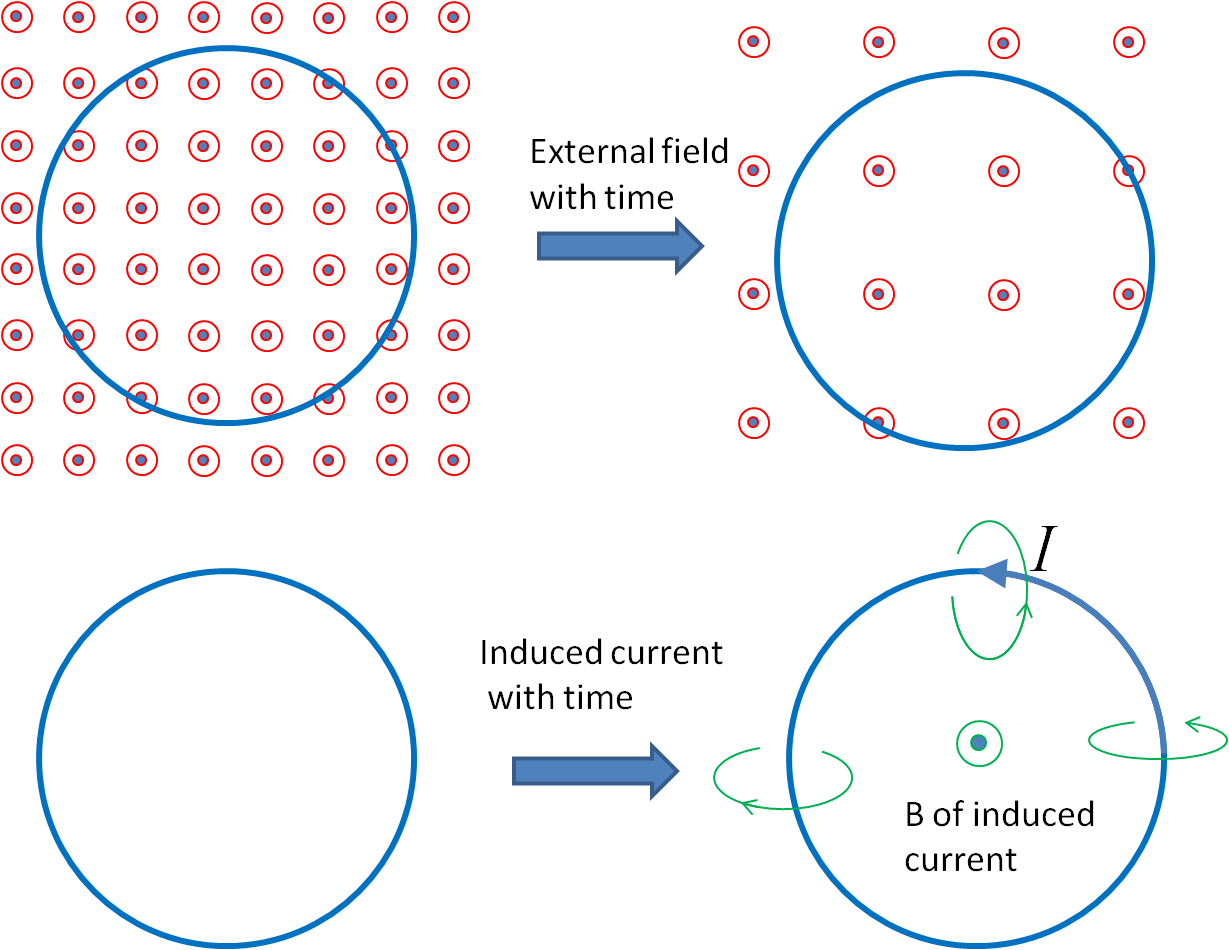Figure 41.3.19. Direction of the induced current by Lenz's law. We use the fact that the magnetic field of the induced current opposes the change in the magnetic flux through the space of the loop. The top figures show that the magnetic flux for magnetic field pointed out-of-page is decreasing with time. The bottom figures show the induced current tending to compensate the loss of the magnetic flux by producing magnetic field in the direction in which the new magnetic field can mitigate the loss.

We now utilize Lenz's law to determine the direction of the induced current. Let the direction of magnetic field be pointed up. The operational steps for the application of the Lenz's law for this problem are shown in Figure 41.3.19. We start by noting that, since the magnitude of the magnetic field decreasing with time, the flux of the magnetic field of the type pointed-up would be decreasing with time. Therefore, according to the Lenz's law, the magnetic flux of the magnetic field of the induced current must add to the magnetic flux of the pointed-up type field. That is, the magnetic field of the induced current should be pointed up at points inside the loop. This gives the direction of counter-clockwise for the induced current as looked from above as shown in Figure 41.3.19.